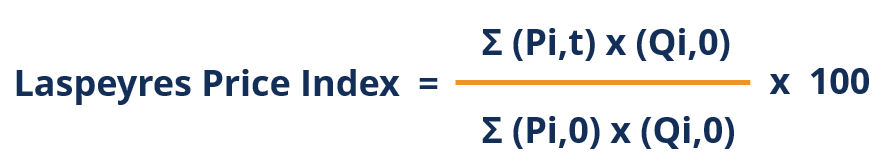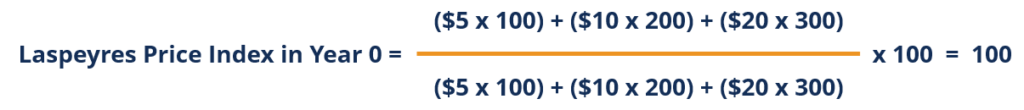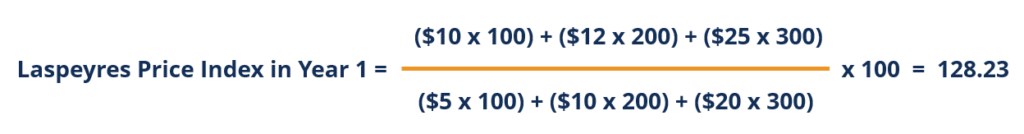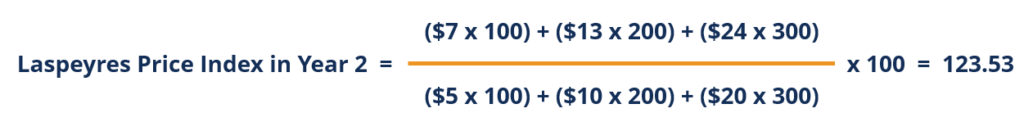# Laspeyres Price Index

Measures the change in the prices of a basket of goods and services relative to a specified base period weighting

## What is the Laspeyres Price Index?

The Laspeyres Price Index is a consumer price index used to measure the change in the prices of a basket of goods and services relative to a specified base period weighting. Developed by German economist Etienne Laspeyres, the Laspeyres Price Index is also called the base year quantity weighted method.### Understanding the Laspeyres Price Index

The Laspeyres Price Index is a price index used to measure the economy’s general price level and cost of living, and to calculate inflation. The index commonly uses a base year figure of 100, with periods of higher price levels shown by an index greater than 100 and periods of lower price levels by indexes lower than 100.

A key differentiator between the Laspeyres Price Index and other indices (Paasche Price Index, Fisher Price Index, etc.) is that it uses weights taken from a base period.

### Formula for the Laspeyres Price Index

The formula for the Laspeyres Price Index is as follows:Where:

• Pi,0 is the price of the individual item at the base period and Pi,t is the price of the individual item at the observation period.
• Qi,0 is the quantity of the individual item at the base period.

Do not be confused by the mathematical notations. The numerator is simply the total expenditures for all items at the observation period using base quantities, and the denominator is the total expenditures for all items at the base period use base quantities. Therefore, the Laspeyres Price Index can be more easily understood when rewritten as follows:### Index Example

The following information regarding the change in the prices and quantities of each individual good in a hypothetical economy is provided. Determine the Laspeyres Price Index for Year 0, Year 1, and Year 2, using Year 0 as the base year.

 Item Year 0 Year 1 Year 2 Good A \$5 \$10 \$7 Good B \$10 \$12 \$13 Good C \$20 \$25 \$24

 Item Year 0 Year 1 Year 2 Good A 100 125 150 Good B 200 225 250 Good C 300 325 350

Using the formula for the Laspeyres Price Index:Therefore, the price indexes were as follows for each year:

• Year 0 (Base Year) = 100
• Year 1 = 128.23
• Year 2 = 123.53

Note that, with this index, the only changes are the prices over the years. The quantities for each good remain the same throughout the years.

The advantages of the index include:

• Easy to calculate and commonly used
• Cheap to construct
• Quantities for future years do not need to be calculated – only base year quantities (weightings) are used
• Presents a meaningful comparison, as changes in the index are attributable to the changes in price

The main disadvantages of the index are that it is upward-biased and tends to overstate price increases (compared to other price indices). Therefore, it tends to overestimate price levels and inflation. This is due to:

1. New goods: More expensive new goods that cause an upward bias in prices.
2. Quality changes: Price increases solely due to quality improvements should not be considered inflation.
3. Substitution: Substituting goods or services that have become relatively cheaper for those that have become relatively more expensive.

### Key Takeaways

The Laspeyres Price Index is one of the most commonly used price indices in measuring the change in the prices of a basket of goods and services relative to a specified base period weighting.

The numerator of the index is simply the total expenditures of all items at the observation period using base period quantities, while the denominator is the total expenditures of all items using base period prices and quantities.

### More Resources

CFI is the official provider of the Financial Modeling and Valuation Analyst (FMVA)™ certification program, designed to transform anyone into a world-class financial analyst.

To keep learning and developing your knowledge of financial analysis, we highly recommend the additional CFI resources listed below:

• Consumption
• Hyperinflation
• Invisible Hand
• Supply and Demand

### Financial Analyst Certification

Become a certified Financial Modeling and Valuation Analyst (FMVA)® by completing CFI’s online financial modeling classes!